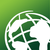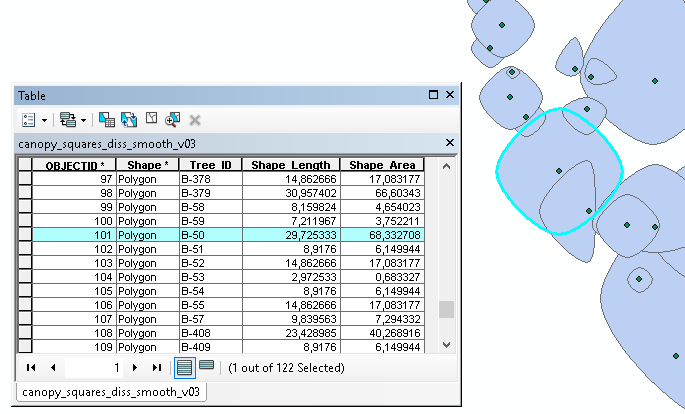# Is it possible to create canopy spreads using precise distances and directions from the stem?

636
14
01-26-2018 03:53 AMby
New Contributor

Hi

Im currently creating Tree Constraints Plans in Arc but having trouble creating "representative canopy spreads". Our surveyors collect a North/South/East/West distance from a central point  and these distances are then used to depict the canopy spread.

We've been creating the canopies by calculating the average size using the measurements above then using this calculation to create a buffer - a nice circle and works very well. However we'd like to get a more representative shape as i'm sure you're aware tree canopies are rarely circular.

My initial thoughts were to place 4 points around the a center point at the distances and directions provided, then create a polygon using these points and finally smooth  the polygon to get an elliptical shape. However this is a long process, especially if we have over 200 trees to work with. That would mean plotting an additional 800 points at specific distances, then digitizing each individual canopy.....there must be a tool or a way of automating the process.

I've attached a couple of examples of the canopies. Example 1 is what we are aiming for example 2 is our current output.

Any ideas or suggestions would be hugely appreciated!

14 RepliesbyEsri Esteemed Contributor

have a look at this code:

``def main():    import arcpy    import os    # output and intermediate fcs    fc_pnt  = r'C:\GeoNet\TreeElipse\tree_data_sample.gdb\tree_data'    fc_pol1 = r'C:\GeoNet\TreeElipse\tree_data_sample.gdb\canopy_squares_v03'    # fc_pol2 = r'C:\GeoNet\TreeElipse\tree_data_sample.gdb\canopy_squares_diss_v03'    fc_pol3 = r'C:\GeoNet\TreeElipse\tree_data_sample.gdb\canopy_squares_diss_smooth_v03'    # flds    fld_n = 'Spread___N'    fld_e = 'Spread___E'    fld_s = 'Spread___S'    fld_w = 'Spread___W'    fld_id = 'Tree_ID'    # some settings    sr = arcpy.Describe(fc_pnt).spatialReference    arcpy.env.overwriteOutput = True    # generate the points and the NESW values into a list    flds = ('SHAPE@', fld_n, fld_e, fld_s, fld_w, fld_id)    feats = [[r.firstPoint, r, r, r, r, r] for r in arcpy.da.SearchCursor(fc_pnt, flds)]    # step 1: create polygons using straight lines    polygons = {}    for feat in feats:        pnts = []        tree_id = feat        lst_apply = [(0, 1), (1, 0), (0, -1), (-1, 0)]        for i in range(1, len(feat)-1):            tpl = lst_apply[i-1]            pnt = arcpy.Point(feat.X+tpl*feat[i], feat.Y+tpl*feat[i])            pnts.append(pnt)        pnts.append(pnts)        polygon = arcpy.Polygon(arcpy.Array(pnts), sr)        polygons[tree_id] = polygon    # create the featureclass    ws, fc_pol1_name = os.path.split(fc_pol1)    arcpy.CreateFeatureclass_management(ws, fc_pol1_name, "POLYGON", None, None, None, sr)    # add the ID field    arcpy.AddField_management(fc_pol1, fld_id, "TEXT", None, None, 10)    # store the polygons from step 1    flds1 = (fld_id, 'SHAPE@')    with arcpy.da.InsertCursor(fc_pol1, flds1) as curs:        for tree_id, polygon in polygons.items():            curs.insertRow((tree_id, polygon, ))    # step 2: Use dissolve to union polygons with overlap##    arcpy.Dissolve_management(in_features=fc_pol1, out_feature_class=fc_pol2,##                              dissolve_field="", statistics_fields="",##                              multi_part="SINGLE_PART", unsplit_lines="DISSOLVE_LINES")    # step3: use the smooth tool (Advanced license required) to smooth the polygons    # use BEZIER_INTERPOLATION to force the output line to go through the input vertices    arcpy.SmoothPolygon_cartography(in_features=fc_pol1, out_feature_class=fc_pol3,                                    algorithm="BEZIER_INTERPOLATION", tolerance="0 Meters",                                    endpoint_option="FIXED_ENDPOINT", error_option="NO_CHECK")if __name__ == '__main__':    main()‍‍‍‍‍‍‍‍‍‍‍‍‍‍‍‍‍‍‍‍‍‍‍‍‍‍‍‍‍‍‍‍‍‍‍‍‍‍‍‍‍‍‍‍‍‍‍‍‍‍‍‍‍‍‍‍‍‍‍‍‍‍‍‍‍‍‍‍‍‍‍‍‍‍‍‍‍‍‍‍‍‍‍‍‍‍‍‍‍‍‍‍‍‍‍‍‍‍‍‍‍‍‍‍‍‍‍‍‍‍‍‍‍‍‍‍‍‍‍‍‍‍‍‍‍‍‍‍‍‍‍‍``

In this case no dissolving is applied and for each tree the Tree_ID is preserved:BTW you tree with ID "1694" has 0 values for all four directions.

I have attached the FGDB with the newly created featureclasses.New Contributor II

xander_bakker‌ Thanks for that. It works perfect. Exactly what i was after.New Contributor II

Dan_Patterson‌ I did try and spatial join the point data onto the single polygons. However there seems to be alot of overlap, therefore the data wasn't joining accurately.New Contributor

To ask an incredibly basic question - where are you running that code?  As a script within a new tool in the user toolbox?byMVP Esteemed Contributor

a couple of your desired shapes are 'super-ellipses' (b-axis not centered on a-axis).

how strict is the requirement for the shape?